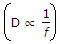# Electronics and Communication Engineering - Electromagnetic Field Theory

1.

When the phase velocity of an EM wave depends on frequency in any medium, the phenomenon is called

 A. scattering B. polarization C. absorption D. dispersion

Explanation:

No answer description available for this question. Let us discuss.

2.

A hertzian dipole has a radiation pattern with

 A. a null in the direction of the dipole B. a null in the plane perpendicular to the dipole C. a peak in the direction of the dipole D. none of the above

Explanation:

No answer description available for this question. Let us discuss.

3.

Circular polarized waves result when

 A. magnitudes are the same B. phases are the same C. magnitudes are same and phase difference is 90° D. magnitudes are same and phase difference is zero

Explanation:

No answer description available for this question. Let us discuss.

4.

Permeance is analogue to

 A. reluctance B. conductance C. capacitance D. resistance

Explanation:

No answer description available for this question. Let us discuss.

5.

Skip distance (D) is

 A. (D ∝ f) B.C. D = f D. D < f